﻿ Multiply
Liquid Studio Documentation
Data Mapping / Data Mapper Functions / By Category / Maths / Multiply
In This Topic
Multiply
In This Topic

Multiply
Maths
##### Icon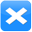##### Description
Multiply two numeric values
##### Inputs
 Value 1 First value to multiply Value 2 Second value to multiply
##### Outputs
 Result Result of multiplying value 1 and value 2
##### Properties
 None -

##### Usage

The Data Mapper Maths Multiply function allows you to carry out multiplication on input values. You can use the Multiply function with input source data or in conjunction with other arithmetic functions in the Maths section. To apply the Maths Multiply function, use the following process:

Create a new Data Mapper file, dragging your XML data source and targets into the editor area. For this example we are using payroll_source.xsd, an XML Schema Definition inferred from the following source:

Source XML
Copy Code
```<payroll>
<employee payroll_id="126">
<rate>9.45</rate>
<hours>35</hours>
<bonus>50.00</bonus>
<funding>350</funding>
</employee>
<employee payroll_id="129">
<rate>10.50</rate>
<hours>40</hours>
<bonus>150.00</bonus>
<funding>500</funding>
</employee>
<employee payroll_id="130">
<rate>8.90</rate>
<hours>35</hours>
<bonus>100.00</bonus>
<funding>400</funding>
</employee>
<employee payroll_id="133">
<rate>11.20</rate>
<hours>40</hours>
<bonus>200.00</bonus>
<funding>600</funding>
</employee>
</payroll>
```

For our target schema we will be using payroll_target.xsd, inferred from the following XML:

Target XML
Copy Code
```<wages>
<payee>
<ref>122</ref>
<result type="wage">500.50</result>
</payee>
<payee>
<ref>125</ref>
<result type="wage">475.40</result>
</payee>
<payee>
<ref>126</ref>
<result type="wage">600.80</result>
</payee>
</wages>
```

The data models payroll data for temporary staff within an organisation. The output is designed to capture some value calculated from the source, using the "type" attribute to indicate what the calculation result represents. Let's assume that we want to calculate the basic weekly wage for each staff member, not including their bonus. To calculate this we will need to multiply the hourly rate by the number of hours worked. Here is the Data Mapper with Source and Target imported: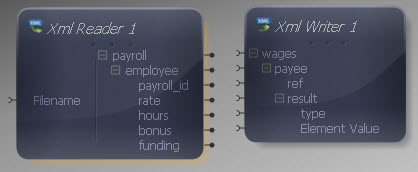Drag the Maths Multiply function from the Component Palette into the mapping area: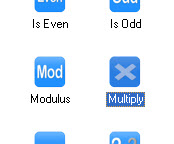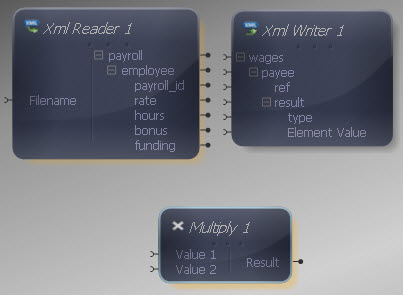The Multiply component has two inputs, representing the values to multiply. Both inputs and output of the Multiply function may be connected to items in the XML Reader/ Writer or other components in the Mapper.

Connect the Multiply inputs to the "rate" and "hours" outputs in the XML Reader.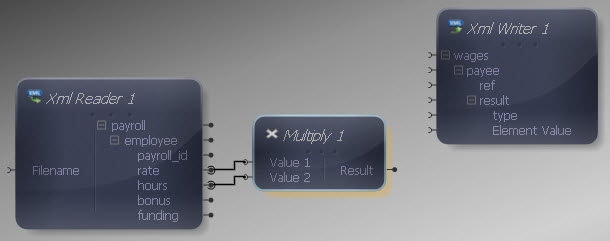Now connect the Multiply output to the Element Value input in the XML Writer, so that the multiplication result is written into the "result" element.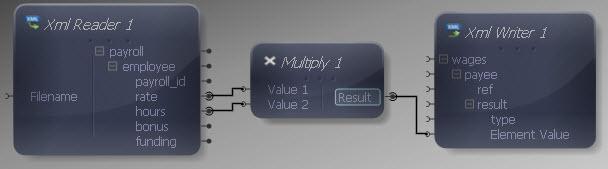Now we need to include the "type" attribute in the source, so add a Constant Value from the Data Type section. Right-click it and choose Show Properties. Choose a String Data Type and enter "wage" as the value.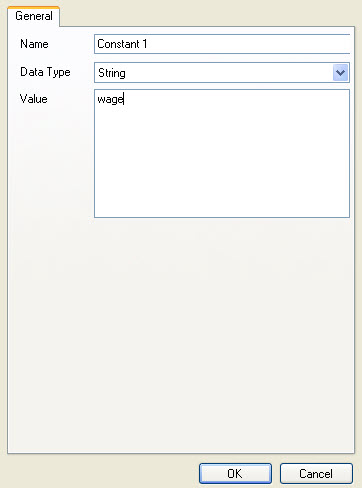Connect the Constant output to the "type" input in the XML Writer.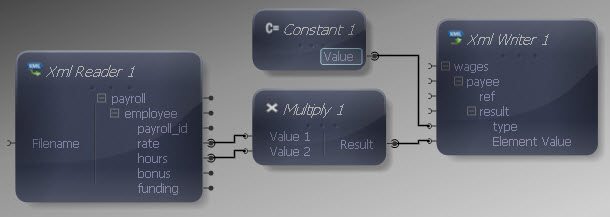Finally let's make the remaining input and output connections. We do not want to map all data items in the source, as we are only interested in certain values. Map "payroll" to "wages", "employee" to "payee" and "payroll_id" to "ref". We don't need to map anything to the "result" element as it will be written out each time the Mapper maps the multiplication result to it.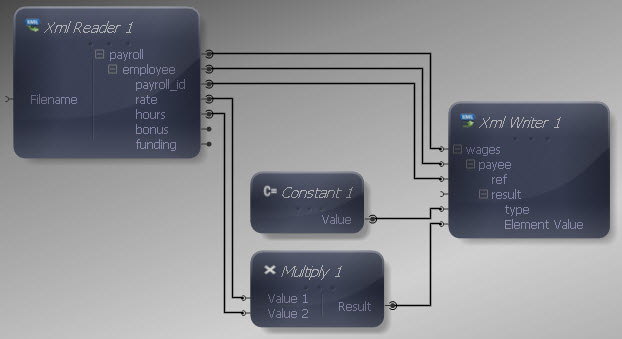We can now execute the transform by pressing Shift-F5 or the Execute button (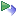). The transform is applied and the file we selected as output opens in the editor:

Output XML
Copy Code
```<wages>
<payee>
<ref>126</ref>
<result type="wage">330.75</result>
</payee>
<payee>
<ref>129</ref>
<result type="wage">420.00</result>
</payee>
<payee>
<ref>130</ref>
<result type="wage">311.50</result>
</payee>
<payee>
<ref>133</ref>
<result type="wage">448.00</result>
</payee>
</wages>
```

The output contains an indicator of the weekly wage for each employee, comprising the result of the multiplication.

This is a scalar function so the standard rules apply if multiple values are presented to one or more inputs.